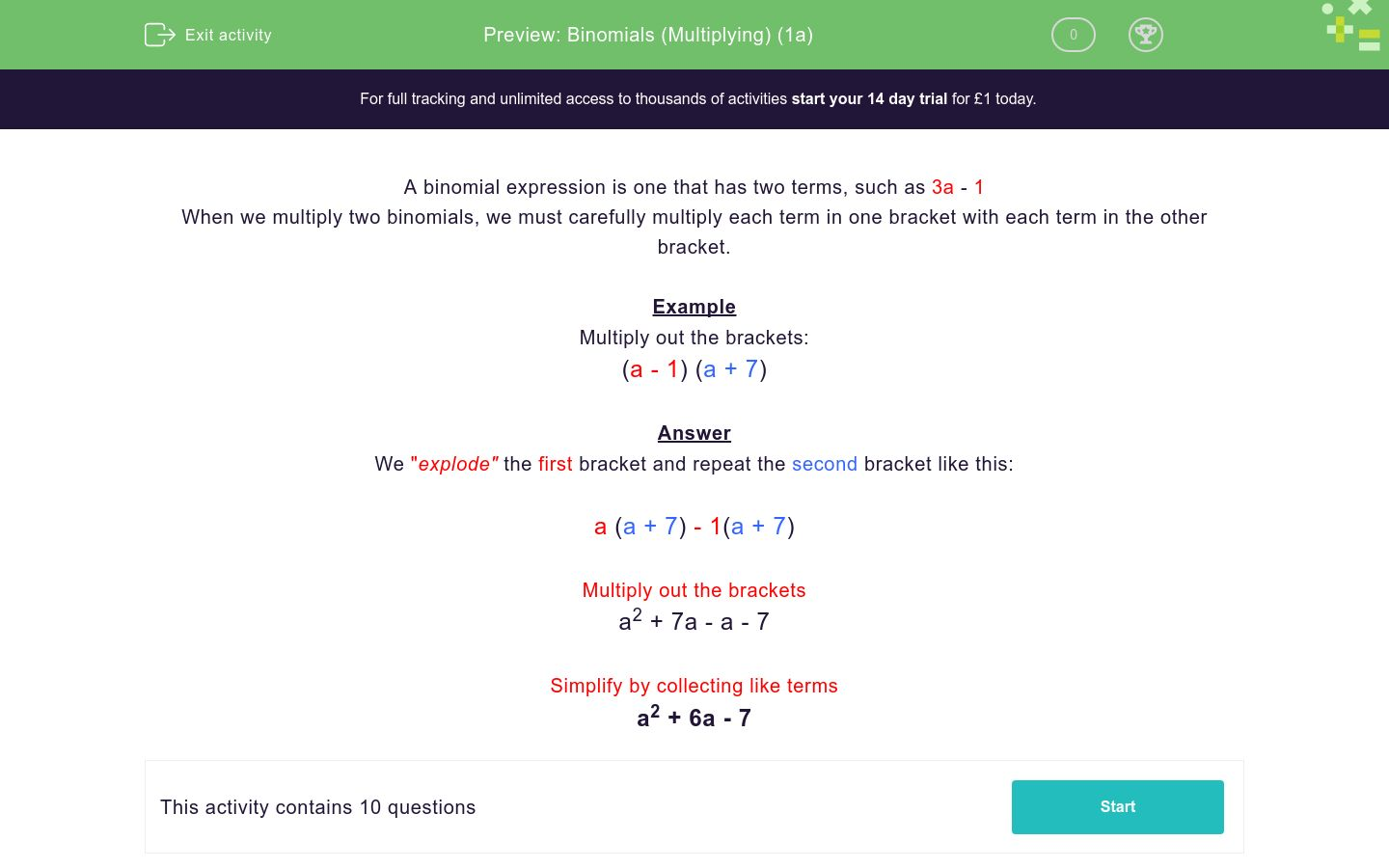# Binomials (Multiplying) (1a)

In this worksheet, students multiply together two binomial expressions.Key stage:  KS 4

Curriculum topic:  Algebra

Difficulty level:### QUESTION 1 of 10

A binomial expression is one that has two terms, such as 3a - 1

When we multiply two binomials, we must carefully multiply each term in one bracket with each term in the other bracket.

Example

Multiply out the brackets:

(a - 1) (a + 7)

We "explode" the first bracket and repeat the second bracket like this:

a (a + 7) - 1(a + 7)

Multiply out the brackets

a2 + 7a - a - 7

Simplify by collecting like terms

a2 + 6a - 7

Multiply out the brackets:

(a + 2) (a + 1)

a2+2a +1

2a2+2a +1

a2+3a +1

a2+3a +2

Multiply out the brackets:

(a + 2) (a + 3)

a2+2a +6

2a2+6a +5

a2+5a +6

a2+6a +5

Multiply out the brackets:

(a + 6) (a + 1)

a2+7a +6

2a2+6a +5

a2+6a +7

a2+6a +5

Multiply out the brackets:

(a + 4) (a - 1)

a2+5a +4

a2+3a -4

4a2+5a -4

a2+4a +3

Multiply out the brackets:

(a + 3) (a - 2)

a2+5a +6

a2+a -6

3a2+5a -6

a2+4a +6

Multiply out the brackets:

(a + 1) (a - 6)

a2+5a -6

a2+a -6

3a2+5a -6

a2-5a -6

Multiply out the brackets:

(a + 2) (a - 4)

a2+2a -8

a2-2a -8

2a2+2a -6

a2-4a -6

Multiply out the brackets:

(a + 4) (a - 4)

a2+8a -16

a2-4a -8

2a2+2a -16

a2-16

Multiply out the brackets:

(a - 4) (a - 4)

a2+8a -16

a2-8a -16

a2-8a +16

a2-4a -16

Multiply out the brackets:

(a - 3) (a - 4)

a2+a -12

a2-a -12

a2-a +7

a2-7a +12

• Question 1

Multiply out the brackets:

(a + 2) (a + 1)

a2+3a +2
• Question 2

Multiply out the brackets:

(a + 2) (a + 3)

a2+5a +6
• Question 3

Multiply out the brackets:

(a + 6) (a + 1)

a2+7a +6
• Question 4

Multiply out the brackets:

(a + 4) (a - 1)

a2+3a -4
• Question 5

Multiply out the brackets:

(a + 3) (a - 2)

a2+a -6
• Question 6

Multiply out the brackets:

(a + 1) (a - 6)

a2-5a -6
• Question 7

Multiply out the brackets:

(a + 2) (a - 4)

a2-2a -8
• Question 8

Multiply out the brackets:

(a + 4) (a - 4)

a2-16
• Question 9

Multiply out the brackets:

(a - 4) (a - 4)

a2-8a +16
• Question 10

Multiply out the brackets:

(a - 3) (a - 4)